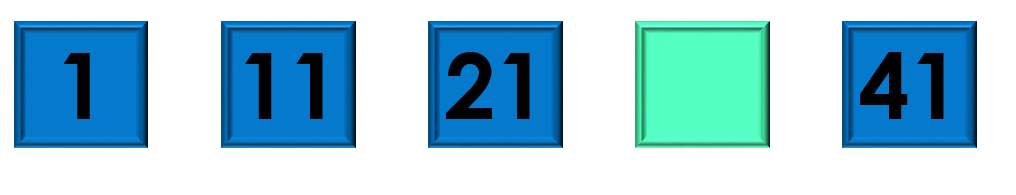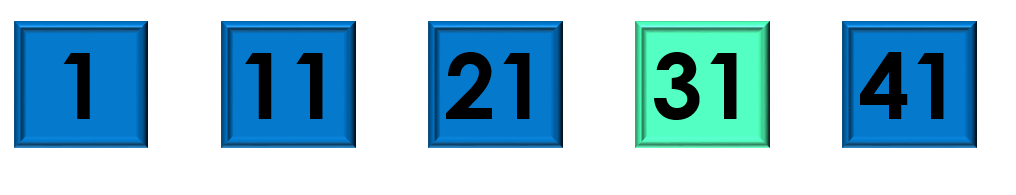1
visibility

Consider the given number pattern and identify the missing number.• A

32

• B

33

• C

31

• D

23

Solution:

Considering the given number pattern.Here, the numbers are increasing.
So, to find the missing number, we first find the rule.

Note that:
1 + 10 = 11
11 + 10 = 21
21 + 10 = 31
31 + 10 = 41

So, the rule is "add 10".Then, the missing number is 31.